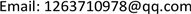﻿ 用吴方法解决染色问题 Solve the Dyeing Problem by Wu’s Method

Pure Mathematics
Vol. 08  No. 05 ( 2018 ), Article ID: 26718 , 5 pages
10.12677/PM.2018.85065

Solve the Dyeing Problem by Wu’s Method

Tingting Miao

School of Mathematics and System Sciences, Beihang University, BeijingReceived: Aug. 13th, 2018; accepted: Aug. 30th, 2018; published: Sep. 6th, 2018ABSTRACT

Dyeing problem is a famous problem in graph theory; the dyeing problem has been solved by Groebner bases method. This text applies the technique of Wu’s method to solve the dyeing problem. The Wu’s method is also called the feature list method; it is a method to deal with polynomial algebra problem proposed by Wu Wenjun in the 1970s. The difference between Wu’s method with Groebner bases method is that Wu’s method completely uses the viewpoint of zero set to deal with the problem, so it is more effective than Groebner bases method in solving dyeing problems; this paper only solves the 3-color problem in the dyeing problem.

Keywords:The Dyeing Problem, Wu’s Method, Zero Set, Groebner Bases Method, The 3-Color Problem1. 引言

${x}_{i}^{3}-1=0,\text{\hspace{0.17em}}\text{\hspace{0.17em}}i=1,2,\cdots ,n$(1.1)

${x}_{i}^{2}+{x}_{i}{x}_{j}+{x}_{j}^{2}=0$(1.2)

2. 预备知识

2.1. 升列的定义

1) $cl\left(F\right)

2) $cl\left(F\right)=cl\left(G\right)=p>0$，但 ${\mathrm{deg}}_{{x}_{p}}\left(F\right)<{\mathrm{deg}}_{{x}_{p}}\left(G\right)$

$A:{A}_{1},{A}_{2},\cdots ,{A}_{r}$

1) $r=1,{A}_{1}\ne 0$

2) $r>1,0，且对任意 $j>i,{A}_{j}red/{A}_{i}$

2.2. 矛盾列的定义

2.3. 基列的定义

$B:{B}_{1},{B}_{2},\cdots ,{B}_{s}$

1) 存在 $j\le \mathrm{min}\left(r,s\right)$，使得 ${A}_{1}~{B}_{1},\cdots ,{A}_{j-1}~{B}_{j-1}$，但 ${A}_{j}\succ {B}_{j}$

2) $s>r$${A}_{1}~{B}_{1},\cdots ,{A}_{r}~{B}_{r}$

2.4. 特征列的定义

2.5. 多项式组的零点分解

3. 主要结论

3.1. 吴方法解决染色问题的过程

$\begin{array}{c}{x}_{i}^{2}+{x}_{i}{x}_{j}+{x}_{j}^{2},\left(i,j\right)\in \left\{\left(1,2\right),\left(1,5\right),\left(1,6\right),\left(2,3\right),\left(2,4\right),\left(2,8\right),\left(3,4\right),\\ \text{\hspace{0.17em}}\text{\hspace{0.17em}}\text{\hspace{0.17em}}\left(3,8\right),\left(4,5\right),\left(4,7\right),\left(5,6\right),\left(5,7\right),\left(6,7\right),\left(7,8\right)\right\}\end{array}$

$\begin{array}{l}CS=\left\{{x}_{1}-{x}_{7},{x}_{2}+{x}_{7}+{x}_{8},{x}_{3}-{x}_{7},{x}_{4}-{x}_{8},{x}_{5}+{x}_{7}+{x}_{8},\\ \text{\hspace{0.17em}}\text{\hspace{0.17em}}\text{\hspace{0.17em}}\text{\hspace{0.17em}}\text{\hspace{0.17em}}\text{\hspace{0.17em}}\text{\hspace{0.17em}}\text{\hspace{0.17em}}\text{\hspace{0.17em}}{x}_{6}-{x}_{8},{x}_{7}^{2}+{x}_{7}{x}_{8}+{x}_{8}^{2},{x}_{8}^{3}-1\right\}\end{array}$Figure 1. The 3-color figure

$zero\left(PS\right)=zero\left(CS/J\right)+zero\left(PS,{I}_{1}\right)+\cdots +zero\left(PS,{I}_{8}\right)$

3.2. 吴方法和Groebner基方法在解决染色问题时的比较

Groebner基方法 在计算过程中会使多项式对增加很快，选取多项式对的无序性也会造成结果不同，项序的选择和终止条件的复杂性与否都会影响计算的效率。吴方法 在计算多项式方程组时效率较高，速度也很快，整体来说在解决染色问题上吴方法比Groebner基方法更快速和有效。

Solve the Dyeing Problem by Wu’s Method[J]. 理论数学, 2018, 08(05): 486-490. https://doi.org/10.12677/PM.2018.85065

1. 1. Adams, W.W. and Loustaunau, P. (2000) An Introduction to Groebner Bases. American Mathematical Society.

2. 2. 张树功, 雷娜, 刘停战. 计算机代数基础[M]. 北京: 科学出版社, 2005.

3. 3. 王东明, 夏壁灿, 李子明. 计算机代数[M]. 北京: 清华大学出版社, 2007.

4. 4. Cox, D., Little, J. and O’Shea, D. (1996) Ideals, Varieties, and Algoithms. 2nd Edition, Springer, New York.

5. 5. Mishra, B. (1993) Algorithmic Algebra. Springer-Verlag, New York. https://doi.org/10.1007/978-1-4612-4344-1

6. 6. Cox, D., Little, J. and O’Shea, D. (1997) Ideals, Varieties and Algorithms. Sprin-ger-Verlag, New York.

7. 7. Ritt, J.F. (1950) Differential Algebra. American Mathematical Society, New York. https://doi.org/10.1090/coll/033

8. 8. Wu, W.-T. (2000) Mathematics Mechanization. Science Press and Kluwer Academic Publish-ers.